Multiplication of Monomials

Chapter 8 Class 8 Algebraic Expressions and Identities
Concept wise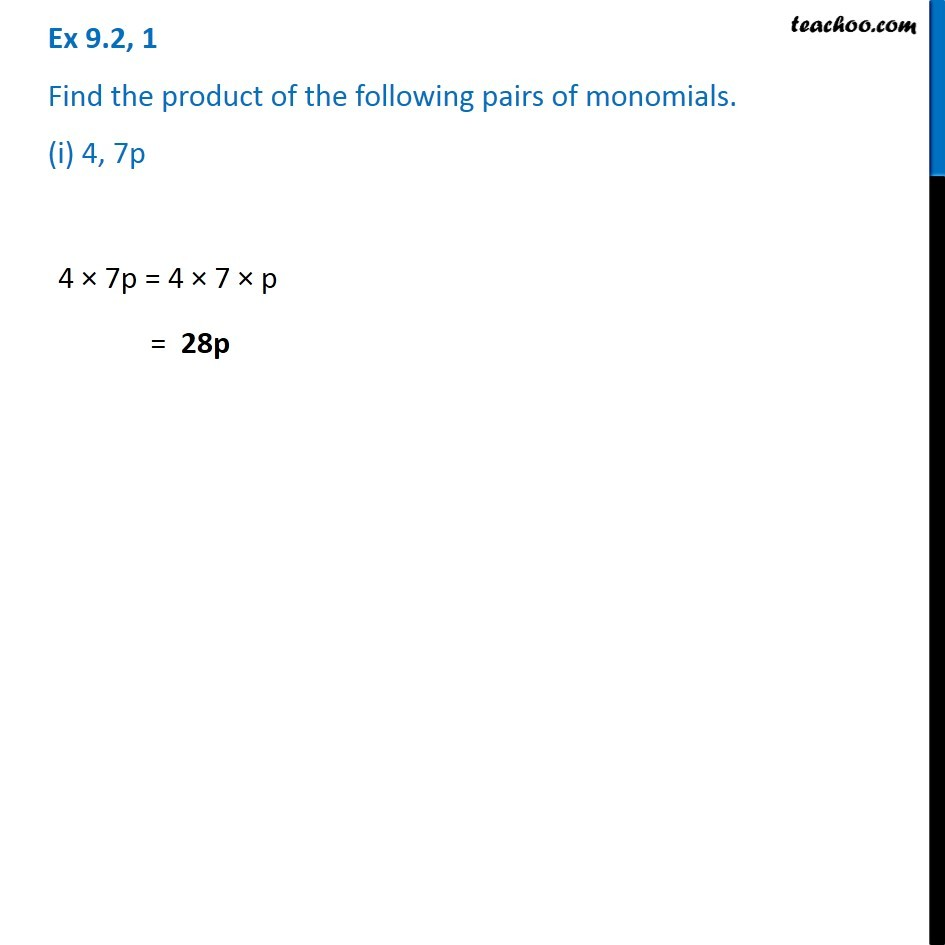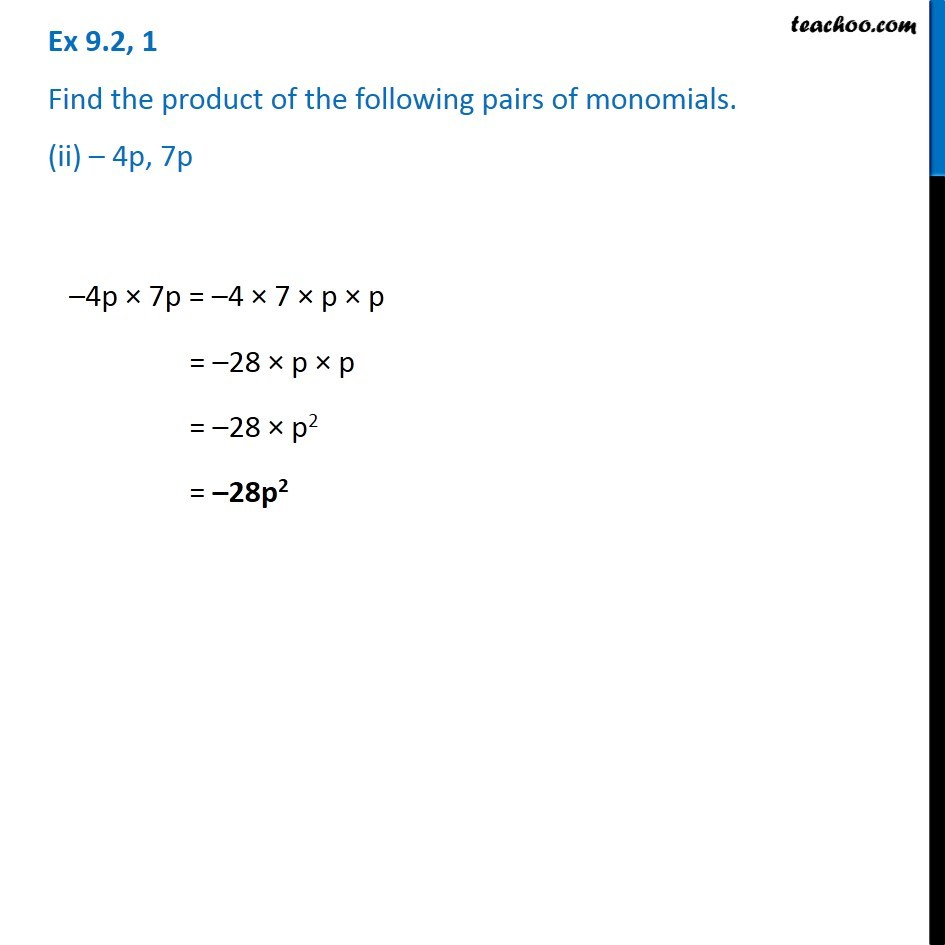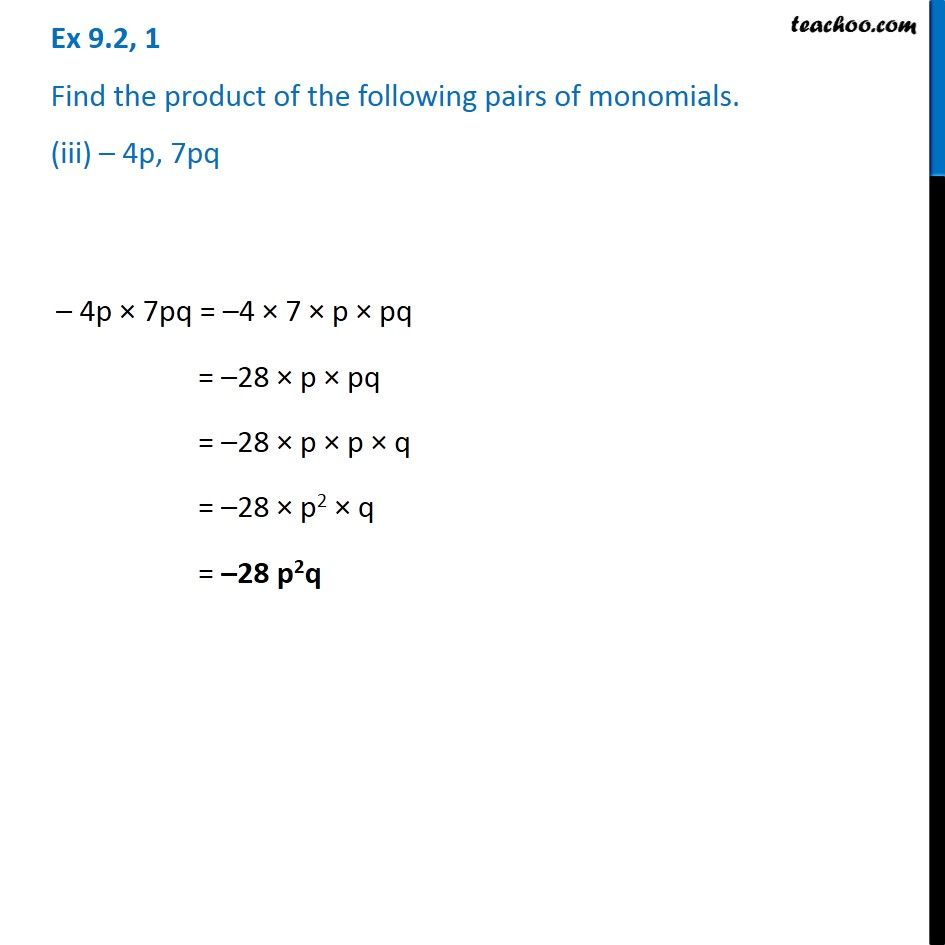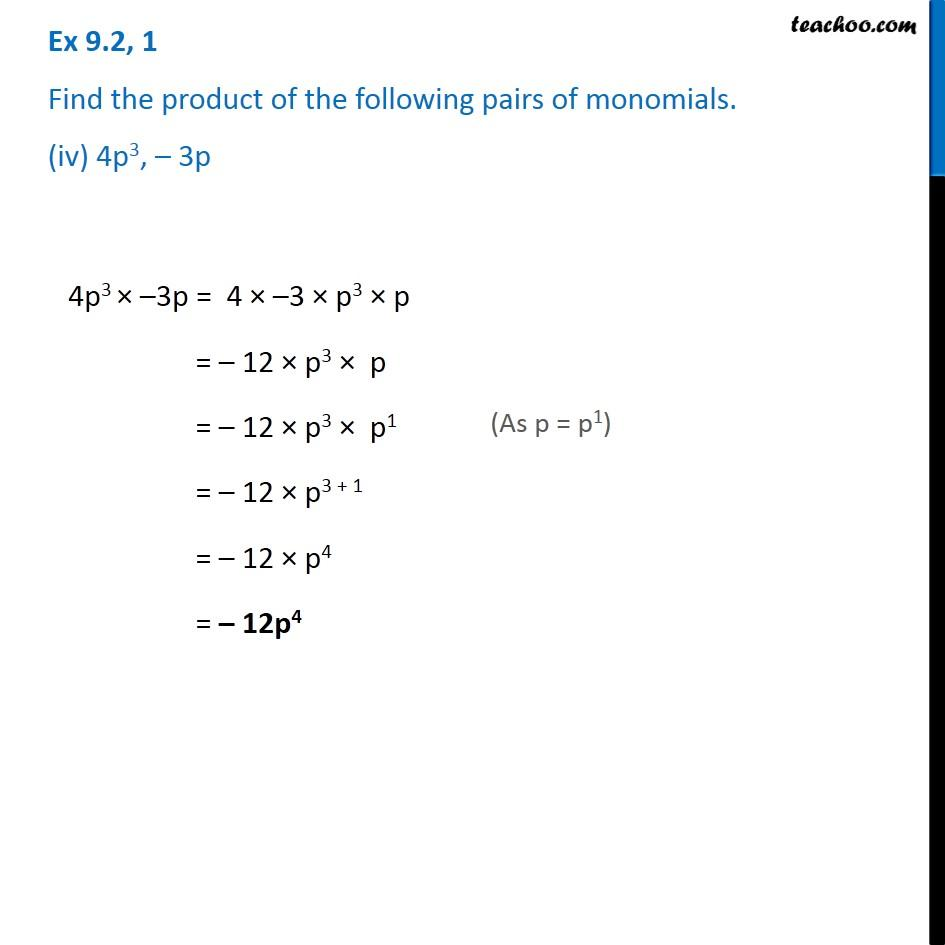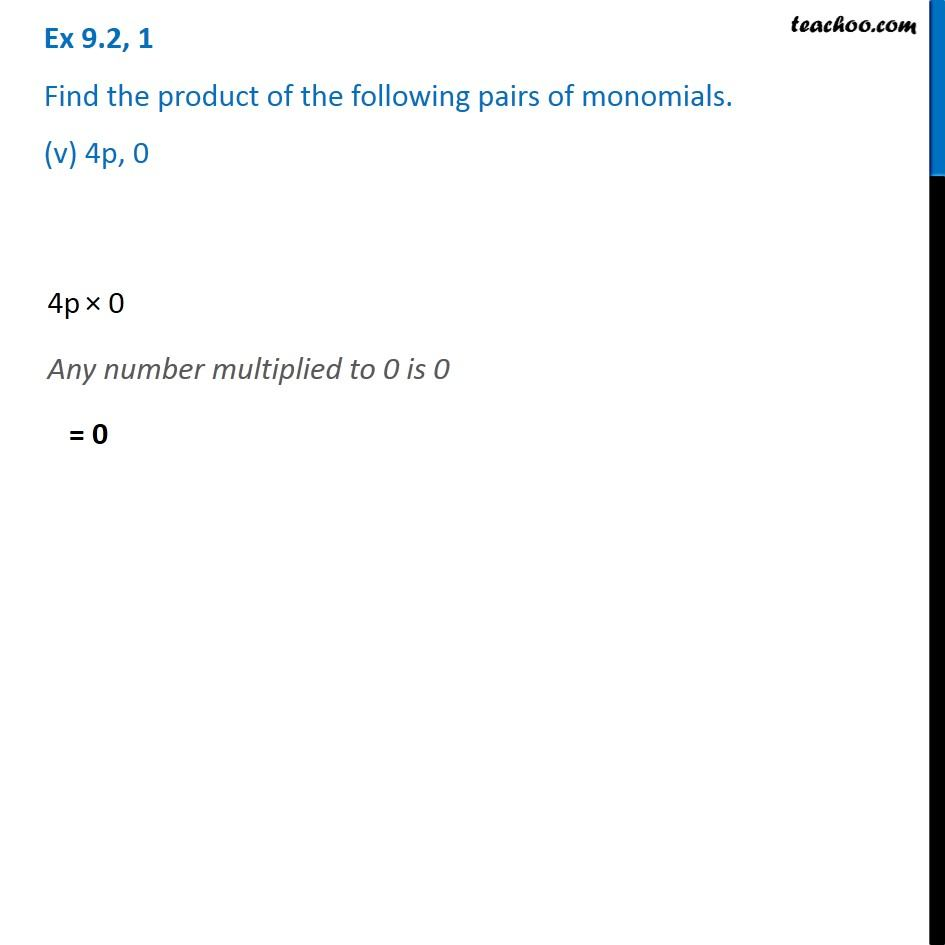Learn in your speed, with individual attention - Teachoo Maths 1-on-1 Class

### Transcript

Ex 8.2, 1 Find the product of the following pairs of monomials. (i) 4, 7p 4 × 7p = 4 × 7 × p = 28p Ex 8.2, 1 Find the product of the following pairs of monomials. (ii) – 4p, 7p –4p × 7p = –4 × 7 × p × p = –28 × p × p = –28 × p2 = –28p2 Ex 8.2, 1 Find the product of the following pairs of monomials. (iii) – 4p, 7pq – 4p × 7pq = –4 × 7 × p × pq = –28 × p × pq = –28 × p × p × q = –28 × p2 × q = –28 p2q Ex 8.2, 1 Find the product of the following pairs of monomials. (iv) 4p3, – 3p 4p3 × –3p = 4 × –3 × p3 × p = – 12 × p3 × p = – 12 × p3 × p1 = – 12 × p3 + 1 = – 12 × p4 = – 12p4 (As p = p1) Ex 8.2, 1 Find the product of the following pairs of monomials. (v) 4p, 0 4p × 0 Any number multiplied to 0 is 0 = 0